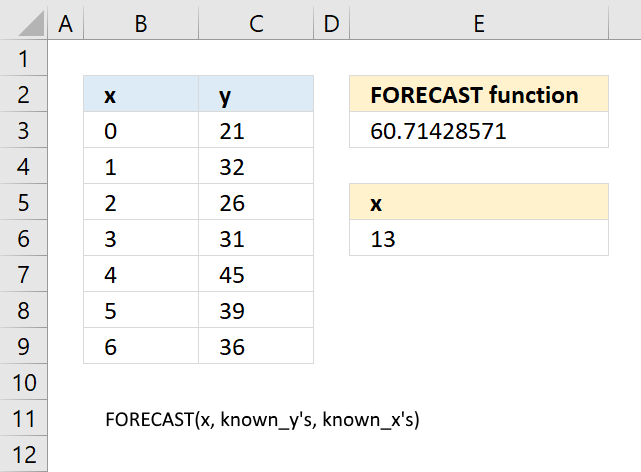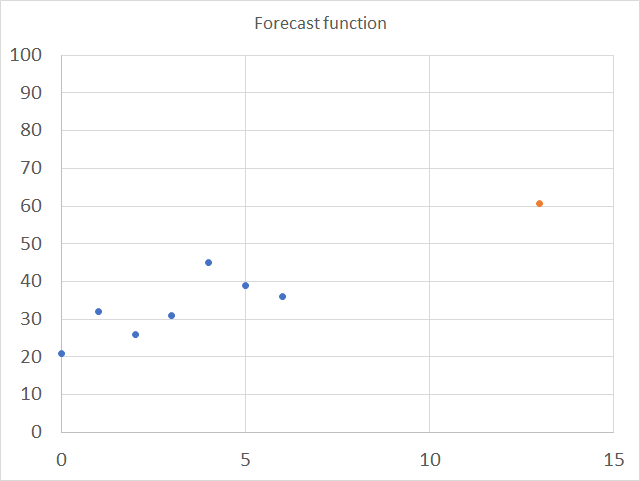Author: Oscar Cronquist Article last updated on October 16, 2018The FORECAST function calculates a value based on existing x and y values using linear regression. Use this function to predict linear trends.

This function is outdated and was replaced with FORECAST.LINEAR function in Excel 2016.

Formula in cell E3:

=FORECAST(E6,C3:C9,B3:B9)

### Excel Function Syntax

FORECAST(x, known_y'sknown_x's)

### Arguments

 x Required. The data point for which you want to predict a value. known_y's Required. Known y points. known_x's Required. Known x points.